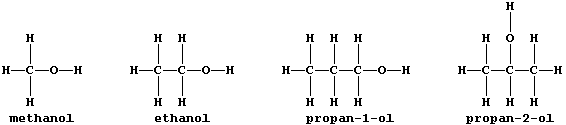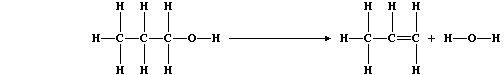```ORGANIC CHEMISTRY: ALCOHOLS
All alcohols contain the hydroxyl functional group, -O-H, and many
belong to an homologous series which has the general formula CnH2n+1OH;
this first Table shows some data for five straight-chain homologues.```
 ` Systematic name ` ```Condensed formula``` ```Boiling pt. / °C``` ``` Density / g cm-ł``` ``` Heat of Combustion (DH) / kJ mol-ą ``` ` Methanol` ` CH3OH` ` 65` ` 0.791` ` -715` ` Ethanol` ` C2H5OH` ` 78` ` 0.798` ` -1370` ` Propan-1-ol` ` C3H7OH` ` 97` ` 0.804` ` -2010` ` Butan-1-ol` ` C4H9OH` ` 118` ` 0.810` ` -2650` ` Pentan-1-ol` ` C5H11OH` ` 138` ` 0.815` ` -3320````1.  Use the data in this Table to state, as precisely as possible, the
pattern between molecular formula and the:
Boiling point _________________________________________________________
_______________________________________________________________________
Heat of combustion ____________________________________________________
_______________________________________________________________________

2.  Extrapolate these data to predict the boiling point and the heat of
combustion for hexan-1-ol. ____________________________________________

3.  Why are propan-1-ol and propan-2-ol considered to be isomeric? ____
_______________________________________________________________________

4.  The symbol equations for the combustion of methanol and propan-1-ol
are as follows:
2CH3OH(g) + 3O2(g) —————————————————————® 2CO2(g) + 4H2O(g)
2C3H7OH(g) + 9O2(g) —————————————————————® 6CO2(g) + 8H2O(g)
(a) Construct the symbol equation for the combustion of pentan-1-ol.
_______________________________________________________________________

(b) How many moles of air are required for the (complete) combustion of
1.0 mole of pentan-1-ol? ______________________________________________
_______________________________________________________________________

(c) Suggest two products, other than carbon dioxide and water, that
might be formed when pentan-1-ol is burnt in a limited supply of air.
_______________________________________________________________________

(d) Calculate the energy released from the combustion of one molecule
of pentan-1-ol (1 mole = 6.022 × 10˛ł particles). _____________________
_______________________________________________________________________

5.  Apart from their use as fuels and as synthetic intermediates,
alcohols are used extensively as non-aqueous solvents; in particular,
and in contrast to water, they dissolve a wide range of covalently
bonded solutes. Although ethanol is drunk socially in beverages (e.g.,
beers and spirits), most alcohols are extremely toxic. Name two human
organs which are damaged by solvent abuse. ____________________________
```

```6.  In industry, large quantities of alkenes are obtained via the
catalytic cracking of petroleum oil fractions. However, the most
convenient method of preparing a small quantity of any alkene is by
catalytic dehydration of the corresponding alcohol; e.g., propene can
be prepared from propan-1-ol using the apparatus shown in the diagram.``````(a) Alcohols can be dehydrated by heating with concentrated sulfuric
acid. Suggest and explain one reason why this dehydrating agent is less
commonly used. ________________________________________________________
_______________________________________________________________________

(b) Explain the purpose of the Bunsen valve. __________________________
_______________________________________________________________________

(c) Suggest what would be observed if, in the above experiment, the
water was replaced with alkaline potassium manganate(VII). ____________
_______________________________________________________________________
_______________________________________________________________________

(d) The equation for this dehydration reaction can be written as:``````Complete this second Table using these bond energies (in kJ mol-ą):
C-C (346); C-O (360); C-H (413); O-H (463); and C=C (610).```
 ` Bonds broken ` ``` Energy absorbed / kJ mol-ą``` ` Bonds formed ` ``` Energy released / kJ mol-ą``` ` 7 C-H ` ` ` ` 6 C-H ` ` ` ` 2 C-C ` ` ` ` 1 C-C ` ` ` ` 1 C-O` ` ` ` 1 C=C` ` ` ` 1 O-H ` ` ` ` 2 O-H ` ` ` ` Total =` ` Total =`
```                                                                    
Calculate the heat energy change (DH) for the above reaction. _________
_______________________________________________________________________

(e) State and explain, using Le Chatelier's Principle, what would be
the effect of high pressure on this dehydration reaction. _____________
_______________________________________________________________________
_______________________________________________________________________

Dr. R. Peters                   Next                     Contents' List```